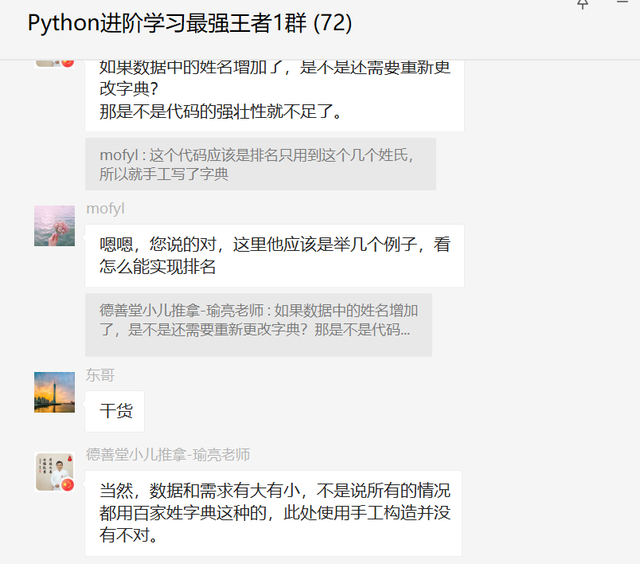# 一、前言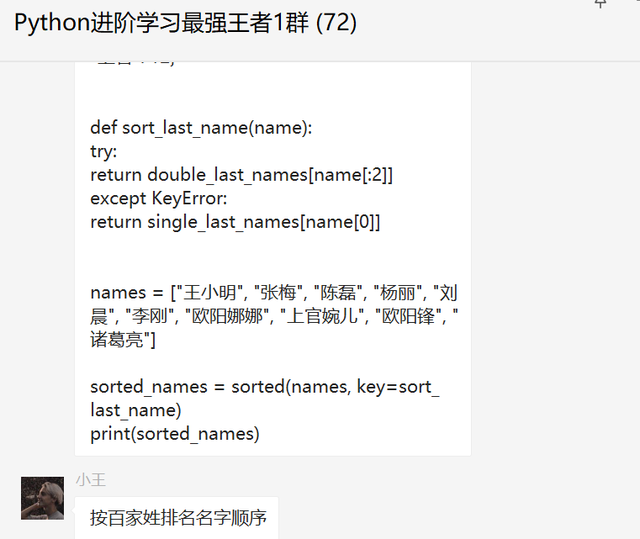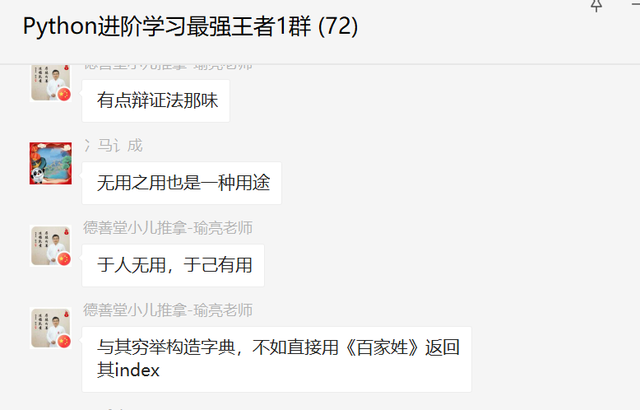# 二、解决过程

``````import re
a = '''

'''
b = re.sub(r'[，。\n]', '', a)
c = {k: v for k, v in enumerate(b)}
print(c)
``````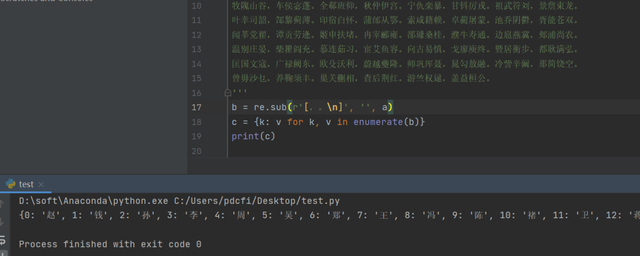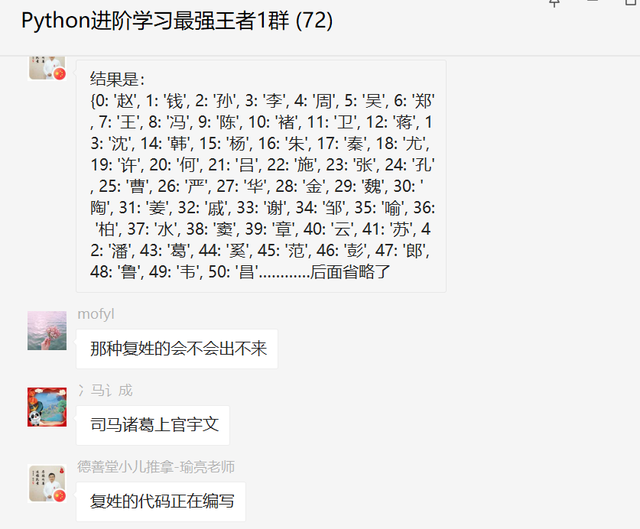``````fx = '''

fx_list = fx.replace("\n", "").split("，")
fx_dict = {k: v for k, v in enumerate(fx_list)}
print(fx_dict)
``````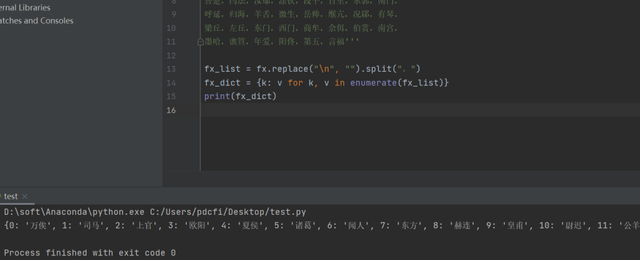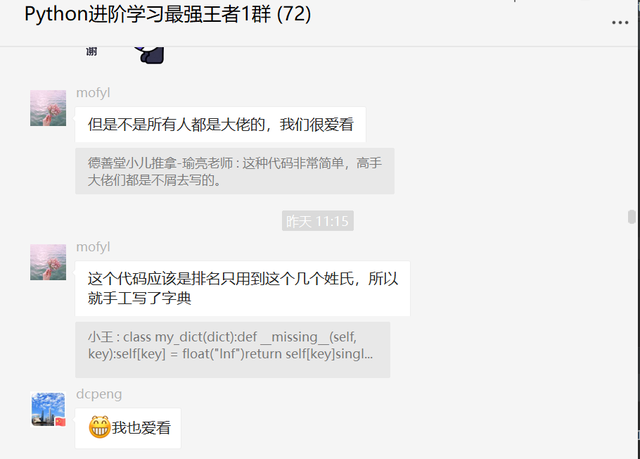``````dict(enumerate(fx.replace('\n', '').split('，')))
``````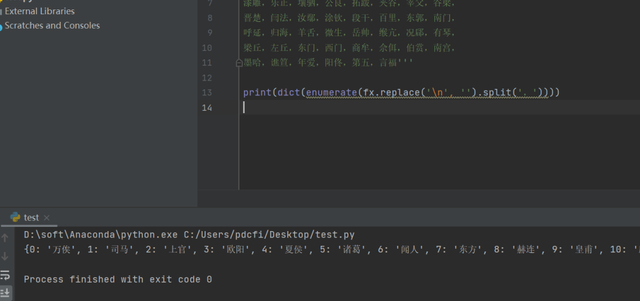# 三、总结